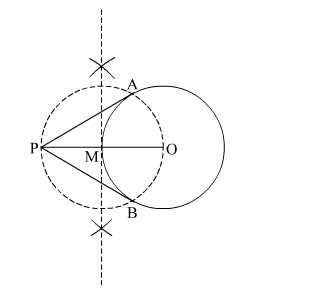# Write the steps of construction to construct the tangents to a circle from an external point.`
Question:

Write the steps of construction to construct the tangents to a circle from an external point.

Solution:

Steps of Construction

Step 1. Draw a circle with O as centre and some radius.

Step 2. Mark a point P outside the circle. Join OP.

Step 3. Draw the perpendicular bisector of OP cutting it at M.

Step 4. Draw another circle with M as centre and radius MP (or OM), to intersect the given circle at the points A and B.

Step 5. Join PA and PB.Here, PA and  PB are the required tangents.